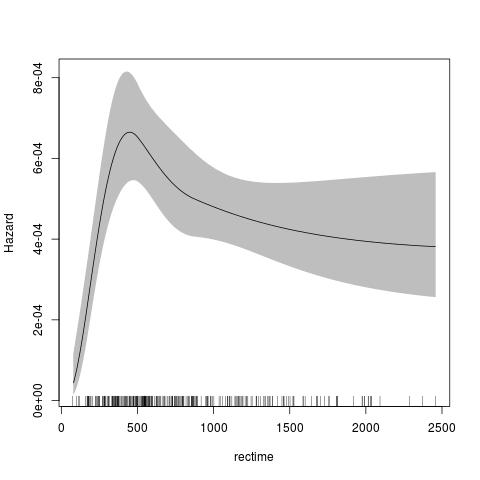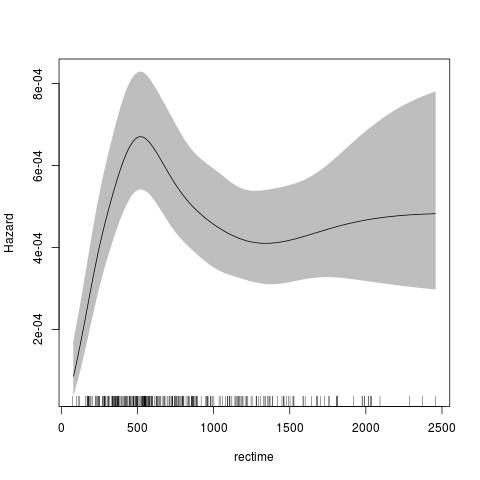# Smooth Survival Models, Including Generalized Survival Models

R implementation of generalized survival models (GSMs), smooth accelerated failure time (AFT) models and Markov multi-state models. For the GSMs, g(S(t|x))=eta(t,x) for a link function g, survival S at time t with covariates x and a linear predictor eta(t,x). The main assumption is that the time effect(s) are smooth . For fully parametric models with natural splines, this re-implements Stata's 'stpm2' function, which are flexible parametric survival models developed by Royston and colleagues. We have extended the parametric models to include any smooth parametric smoothers for time. We have also extended the model to include any smooth penalized smoothers from the 'mgcv' package, using penalized likelihood. These models include left truncation, right censoring, interval censoring, gamma frailties and normal random effects , and copulas. For the smooth AFTs, S(t|x) = S_0(t*eta(t,x)), where the baseline survival function S_0(t)=exp(-exp(eta_0(t))) is modelled for natural splines for eta_0, and the time-dependent cumulative acceleration factor eta(t,x)=\int_0^t exp(eta_1(u,x)) du for log acceleration factor eta_1(u,x). The Markov multi-state models allow for a range of models with smooth transitions to predict transition probabilities, length of stay, utilities and costs, with differences, ratios and standardisation.

## Introduction

This package provides link-based survival models that extend the Royston-Parmar models, a family of flexible parametric models. There are two main classes included in this package:

A. The class stpm2 is an R version of stpm2 in Stata with some extensions, including:

1. Multiple links (log-log, -probit, -logit);

2. Left truncation and right censoring (with experimental support for interval censoring);

3. Relative survival;

4. Cure models (where we introduce the nsx smoother, which extends the ns smoother);

5. Predictions for survival, hazards, survival differences, hazard differences, mean survival, etc;

6. Functional forms can be represented in regression splines or other parametric forms;

7. The smoothers for time can use any transformation of time, including no transformation or log(time).

B. Another class pstpm2 is the implementation of the penalised models and corresponding penalized likelihood estimation methods. The main aim is to represent another way to deal with non-proportional hazards and adjust for potential continuous confounders in functional forms, not limited to proportional hazards and linear effect forms for all covariates. Functional forms can be represented in penalized regression splines (all mgcv smoothers ) or other parametric forms.

## Some examples

The default for the parametric model is to use the Royston Parmar model, which uses a natural spline for the transformed baseline for log(time) with a log-log link.

require(rstpm2)
data(brcancer)
fit <- stpm2(Surv(rectime,censrec==1)~hormon,data=brcancer,df=3)
plot(fit,newdata=data.frame(hormon=0),type="hazard")The default for the penalised model is similar, using a thin-plate spline for the transformed baseline for log(time) with a log-log link. The advantage of the penalised model is that there is no need to specify the knots or degrees of freedom for the baseline smoother.

fit <- pstpm2(Surv(rectime,censrec==1)~hormon,data=brcancer)
plot(fit,newdata=data.frame(hormon=0),type="hazard")# Version 1.4.5

- Fixed a bug in fitting frailty models (introduced in 1.4.4)
- Introduced package tests


# Version 1.4.4

- Fixed a critical bug in the predict function for comparisons of hazards, including type="hr", type="hdiff" and type="marghr" (introduced in 1.4.2).


# Version 1.4.2

- Belatedly started the NEWS.md file
- Update to bbmle (>= 1.0.20) required due to new export from that package
- Possible breaking change: for the predict() functions for stpm2 and pstpm2, the keep.attributes default has changed from TRUE to FALSE. Any code that used predict() and needs the newdata attributes should now add the keep.attributes=TRUE argument. The previous default was noisy.
- Possible breaking change: the derivative of the design matrix with respect to time now defaults to being calculated using log(time); the old calculation can be found using log.time.transform=TRUE. This is expected to provide more accurate gradients, particularly for very small times.
- To this point, the following models are available:
+ stpm2: parametric generalised survival models, possibly with clustered data (Gamma frailties and normal random effects), relative survival, robust standard errors, rich post-estimation and plots.
+ pstpm2: penalised generalised survival models, possibly with clustered data (Gamma frailties and normal random effects), relative survival, robust standard errors, rich post-estimation and plots.
+ aft: parametric accelerated failure time models, with more limited post-estimation and plots.
- Links for the generalised survival models include log-log, -logit, -probit, -log and Aranda-Ordaz.
- Post-estimation for stpm2 and pstpm2 includes:
+ Conditional survival ("surv"), linear predictor ("link"), cumulative hazard ("cumhaz"), hazard ("hazard"), log hazard ("loghazard"), probability density function ("density"), failure ("fail"), hazard ratio ("hr"), survival difference ("sdiff"), hazard difference ("hdiff"), mean survival ("meansurv"), mean survival differences ("meansurvdiff"), mean hazard ratio ("meanhr"), odds ("odds"), odds ratio ("or"), restricted mean survival time ("rmst"), attributable fractions ("af")
+ Marginal survival ("margsurv"), marginal hazard ("marghaz"), attributable fractions ("af"), mean survival ("meanmargsurv")


# Reference manual

install.packages("rstpm2")

1.5.2 by Mark Clements, 9 months ago

https://github.com/mclements/rstpm2

Report a bug at https://github.com/mclements/rstpm2/issues

Browse source code at https://github.com/cran/rstpm2

Authors: Mark Clements [aut, cre] , Xing-Rong Liu [aut] , Benjamin Christoffersen [aut] , Paul Lambert [ctb] , Lasse Hjort Jakobsen [ctb] , Alessandro Gasparini [ctb] , Gordon Smyth [cph] , Patrick Alken [cph] , Simon Wood [cph] , Rhys Ulerich [cph]

Documentation:   PDF Manual

Imports graphics, Rcpp, stats, mgcv, bbmle, fastGHQuad, deSolve, utils, parallel

Depends on methods, survival, splines

Suggests eha, testthat, ggplot2, lattice, readstata13, mstate, scales, survPen